##### Calculus Workbook For Dummies with Online PracticeGeometry tells you how to figure the volumes of simple solids. Integration enables you to calculate the volumes of an endless variety of much more complicated shapes. If you have a round shape with a hole in the center, you can use the washer method to find the volume by cutting that shape into thin pieces. Each slice has a hole in its middle that you have to subtract. There’s nothing to it.

Here you go.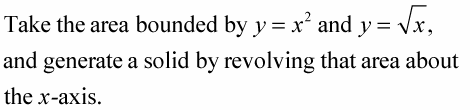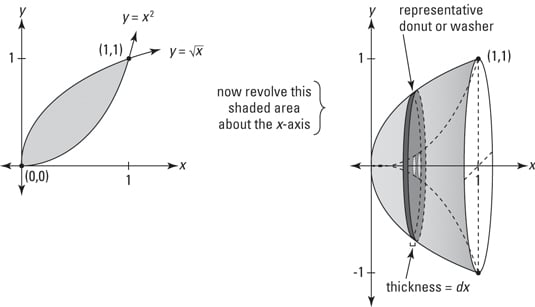A sideways stack of washers — just add up the volumes of all the washers.

Just think: All the forces of the evolving universe and all the twists and turns of your life have brought you to this moment when you are finally able to calculate the volume of this solid — something for your diary. So what’s the volume?

1. Determine where the two curves intersect.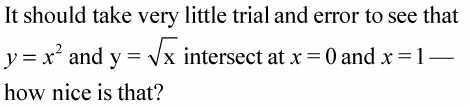So the solid in question spans the interval on the x-axis from 0 to 1.

2. Figure the area of a cross-sectional washer.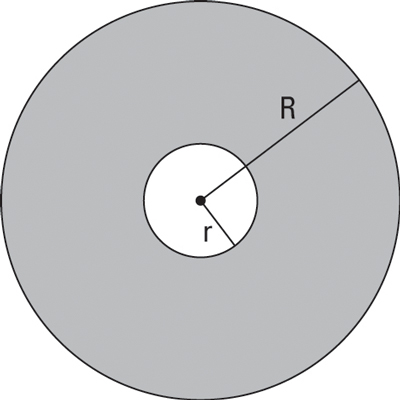In the above figure, each slice has the shape of a washer so its area equals the area of the entire circle minus the area of the hole.

The area of the circle minus the hole is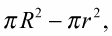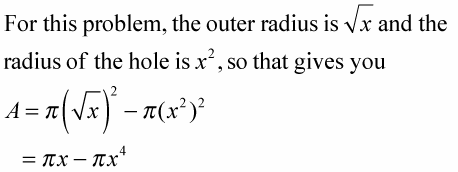3. Multiply this area by the thickness, dx, to get the volume of a representative washer.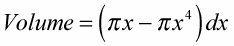4. Add up the volumes of the washers from 0 to 1 by integrating.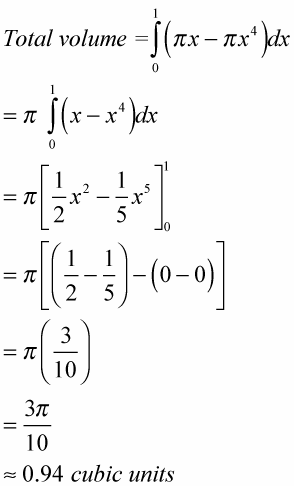Focus on the simple fact that the area of a washer is the area of the entire disk,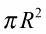minus the area of the hole,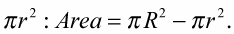When you integrate, you get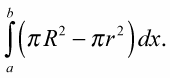This is the same, of course, as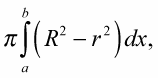which is the formula given in most books. But if you just learn that by rote, you may forget it. You’re more likely to remember how to do these problems if you understand the simple big-circle-minus-little-circle idea.